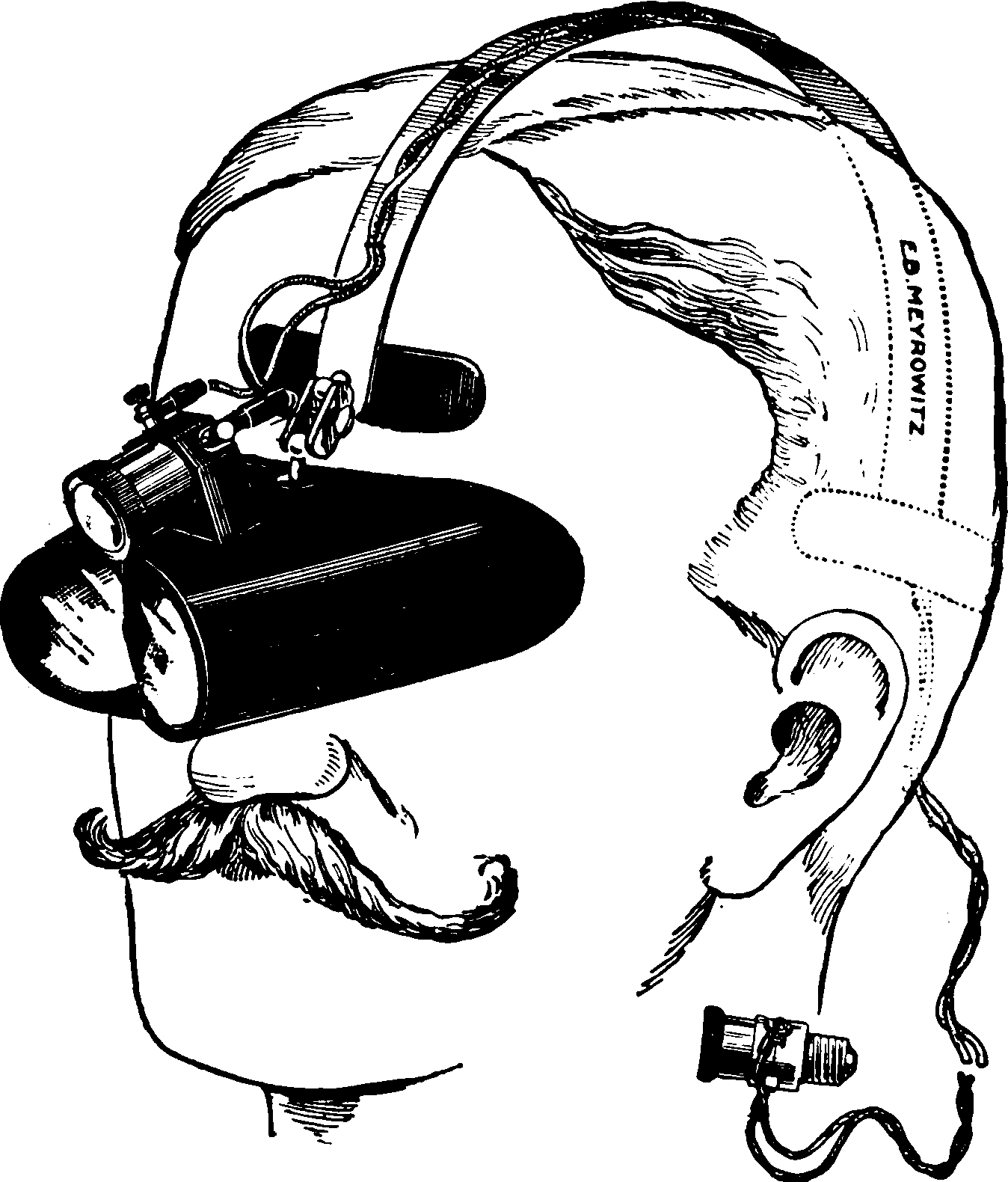# Laplace approximations in inference

## Lightweight uncertainties, especially for heavy neural nets

### Assumed audience:

People who vaguely remember Laplace approximation from calculus maybe but want to know how to make them useful in fancy modern inference

$$\renewcommand{\var}{\operatorname{Var}} \renewcommand{\corr}{\operatorname{Corr}} \renewcommand{\dd}{\mathrm{d}} \renewcommand{\vv}{\boldsymbol{#1}} \renewcommand{\mm}{\mathrm{#1}} \renewcommand{\dist}{\mathcal{#1}} \renewcommand{\rv}{\mathsf{#1}} \renewcommand{\vrv}{\vv{\rv{#1}}} \renewcommand{\disteq}{\stackrel{d}{=}} \renewcommand{\gvn}{\mid} \renewcommand{\Ex}{\mathbb{E}} \renewcommand{\Pr}{\mathbb{P}}$$Second mode? I see no second mode.

Approximating probability distributions by a Gaussian with the same mode. Thanks to limit theorems this is not always a terrible idea, especially since distributions associated with neural networks seem pretty keen to converge to Gaussians in various senses under various useful conditions.

Specifically, we (possibly locally) approximate some posterior density of interest using a Gaussian $p(\theta \mid \mathcal{D}) \approx \mathcal{N}\left(\theta_{\mathrm{MAP}}, \Sigma\right).$ The Laplace trick uses a local curvature estimate to choose the covariance.

I am particularly keen to see it work for probabilistic neural nets, where it is a classic . There are many variants of the technique for different assumptions. We often see this applied to estimating the posterior predictive density, which is usually compact enough to be tractable, but also we can apply it to input uncertainty and even parameters under certain simplifications .

## Basic

In classic Laplace approximation, we assume that the parameters of our model have a Gaussian distribution (independently why not) both a prior and a posteriori. Specifically we then attempt to approximate these using local curvature of the likelihood function at some maximum a posteriori estimate.

⚠️ The next bit needs a do-over. I completely lost track of the prior and the hyperparameters.

Writing that in symbols, the basic idea is that we hold $$x \in \mathbb{R}^{n}$$ fixed and use the Jacobian matrix $$J(x):=\left.\nabla_{\theta} f(x ; \theta)\right|_{\theta_{\mathrm{MAP}}} \in \mathbb{R}^{d \times n}$$, to the network as $f(x ; \theta) \approx f\left(x ; \theta_{\mathrm{MAP}}\right)+J(x)^{\top}\left(\theta-\theta_{\mathrm{MAP}}\right)$ where the variance is now justified using a Taylor expansion and some smoothness assumptions on $$f$$. Under this approximation, since $$\theta$$ is by assumption Gaussian, $$\theta \sim \mathcal{N}\left(\theta_{\mathrm{MAP}}, \Sigma\right)$$, it follows that the marginal distribution over the network output $$f(x)$$ is also Gaussian, given by $f(x) \mid x, \mathcal{D} \sim \mathcal{N}\left(f\left(x ; \theta_{\mathrm{MAP}}\right), J(x)^{\top} \Sigma J(x)\right).$ For more on this, see e.g. . It can be essentially a gratis Laplace approximation in the sense that if I have fit the networks I can already calculate those Jacobians so I am probably 1 line of code away from getting some kind of uncertainty estimate.

I have no particular guarantees that it is well calibrated — the true posterior is almost surely not Gaussian; We need to persuade ourselve the approximation is fit for our current purposes. In other words, this is a variational approximation and it has the usual problems of variational inference.

## Learnable Laplace approximations

NB ⚠️⛔️☣️: This sections is vague half-arsed notes of dubious accuracy. I have not had time to make it into a proper section.

Agustinus Kristiadi and team have created various methods for low-overhead neural uncertainty quantification via Laplace approximation that have greater flexibility for adaptively choosing the type and manner of approximation. See, e.g. Painless Uncertainty for Deep Learning and their papers .

Kristiadi, Hein, and Hennig (2021) generalises to learnable uncertainty to, for example, allow the distribution to reflect uncertainty about datapoints drawn outside the training distribution. They define an augmented Learnable Uncertainty Laplace Approximation (LULA) network $$\tilde{f}$$ with more parameters $$\tilde{\theta}=\theta_{\mathrm{MAP}}, \hat{\theta}.$$

Let $$f: \mathbb{R}^{n} \times \mathbb{R}^{d} \rightarrow \mathbb{R}^{k}$$ be an $$L$$-layer neural network with a MAP-trained parameters $$\theta_{\text {MAP }}$$ and let $$\widetilde{f}: \mathbb{R}^{n} \times \mathbb{R}^{\widetilde{d}} \rightarrow \mathbb{R}^{k}$$ along with $$\widetilde{\theta}_{\text {MAP }}$$ be obtained by adding LULA units. Let $$q(\widetilde{\theta}):=\mathcal{N}\left(\tilde{\theta}_{\mathrm{MAP}}, \widetilde{\Sigma}\right)$$ be the Laplace-approximated posterior and $$p\left(y \mid x, \mathcal{D} ; \widetilde{\theta}_{\mathrm{MAP}}\right)$$ be the (approximate) predictive distribution under the LA. Furthermore, let us denote the dataset sampled i.i.d. from the data distribution as $$\mathcal{D}_{\text {in }}$$ and that from some outlier distribution as $$\mathcal{D}_{\text {out }}$$, and let $$H$$ be the entropy functional. We construct the following loss function to induce high uncertainty on outliers while maintaining high confidence over the data (inliers): $\begin{array}{rl} \mathcal{L}_{\text {LULA }}\left(\widetilde{\theta}_{\text {MAP }}\right)&:=\frac{1}{\left|\mathcal{D}_{\text {in }}\right|} \sum_{x_{\text {in }} \in \mathcal{D}_{\text {in }}} H\left[p\left(y \mid x_{\text {in }}, \mathcal{D} ; \widetilde{\theta}_{\text {MAP }}\right)\right] \\ &-\frac{1}{\left|\mathcal{D}_{\text {out }}\right|} \sum_{x_{\text {out }} \in \mathcal{D}_{\text {out }}} H\left[p\left(y \mid x_{\text {out }}, \mathcal{D} ; \widetilde{\theta}_{\text {MAP }}\right)\right] \end{array}$ and minimize it w.r.t. the free parameters $$\widehat{\theta}$$.

I am assuming that by the entropy functional they mean the entropy of the normal distribution, $H(\mathcal{N}(\mu, \sigma)) = {\frac {1}{2}}\ln \left((2\pi \mathrm {e} )^{k}\det \left({\boldsymbol {\Sigma }}\right)\right)$ but this looks expensive due to that determinant calculation in a (large) $$d\times d$$ matrix. Or possibly they mean some general entropy with respect to some density $$p$$, $H(p)=\mathbb{E}_{p}\left[-\log p( x)\right]$ which I suppose one could estimate as $H(p)=\frac{1}{N}\sum_{i=1}^N \left[-\log p(x_i)\right]$ without taking that normal Laplace approximation at this step, if we could find the density, and assuming the $$x_i$$ were drawn from it.

The result is an unusual, hybrid fitting procedure that requires two loss functions and which feels a little ad hoc, but maybe it works?

## By stochastic weight averaging

A Quasi-Bayesian extension of a gradient descent trick called Stochastic Weight Averaging . AFAICT it precludes using a prior? Any may not actually be a Laplace approximation per se, just some other approximation that is also a Gaussian.

## For model selection

NB ⚠️⛔️☣️: This sections is vague half-arsed notes of dubious accuracy.

We can estimate marginal likelihood with respect to hyperparameters by Laplace approximation, apparently. See Immer et al. (2021).

## In function spaces

Where the Laplace approximations are Gaussian Processes over some index space. TBC. See Piterbarg and Fatalov (1995);Wacker (2017);Alexanderian et al. (2016);Alexanderian (2021) and possibly Solin and Särkkä (2020) and possibly the INLA stuff.

## INLA

Integrated nested Laplace approximation leverages the GP-as-SDE idea to generalise Matérn-type covariances to interesting domains and non-stationarity.